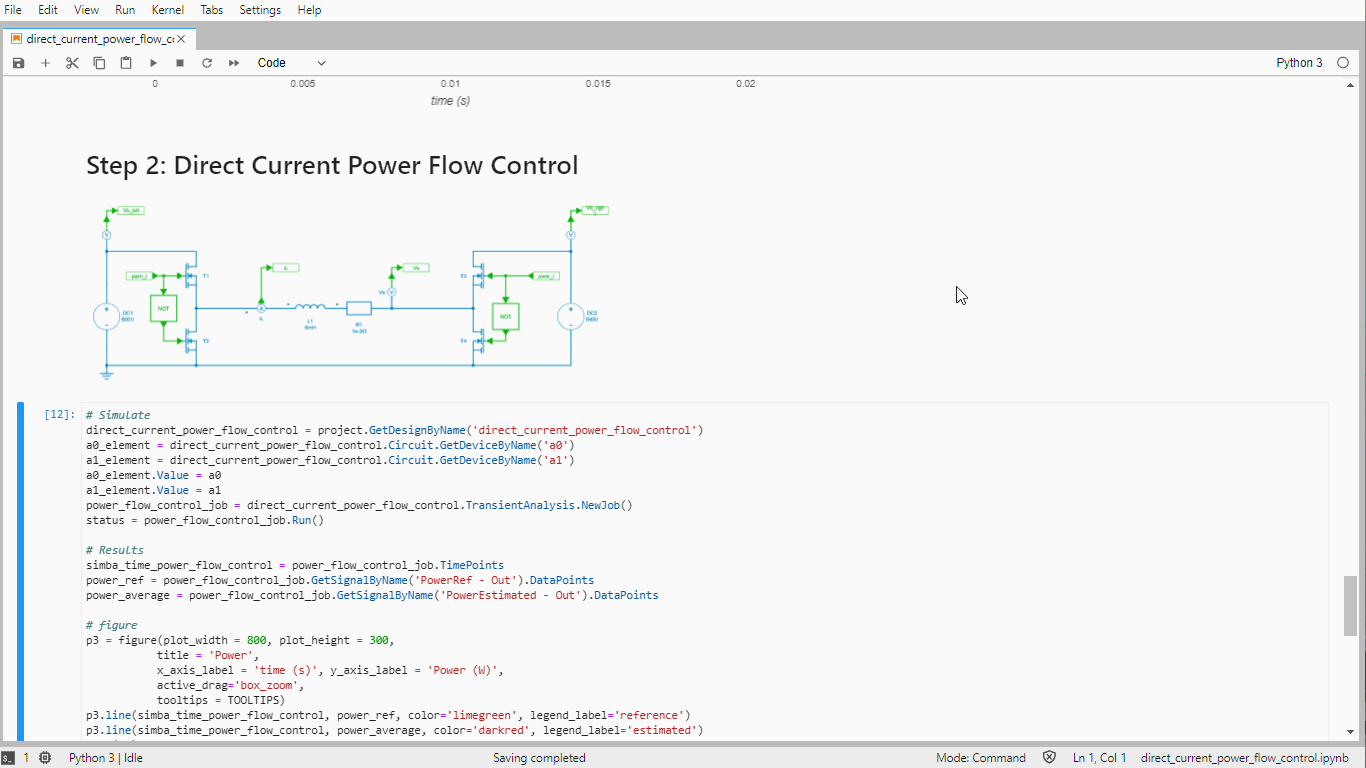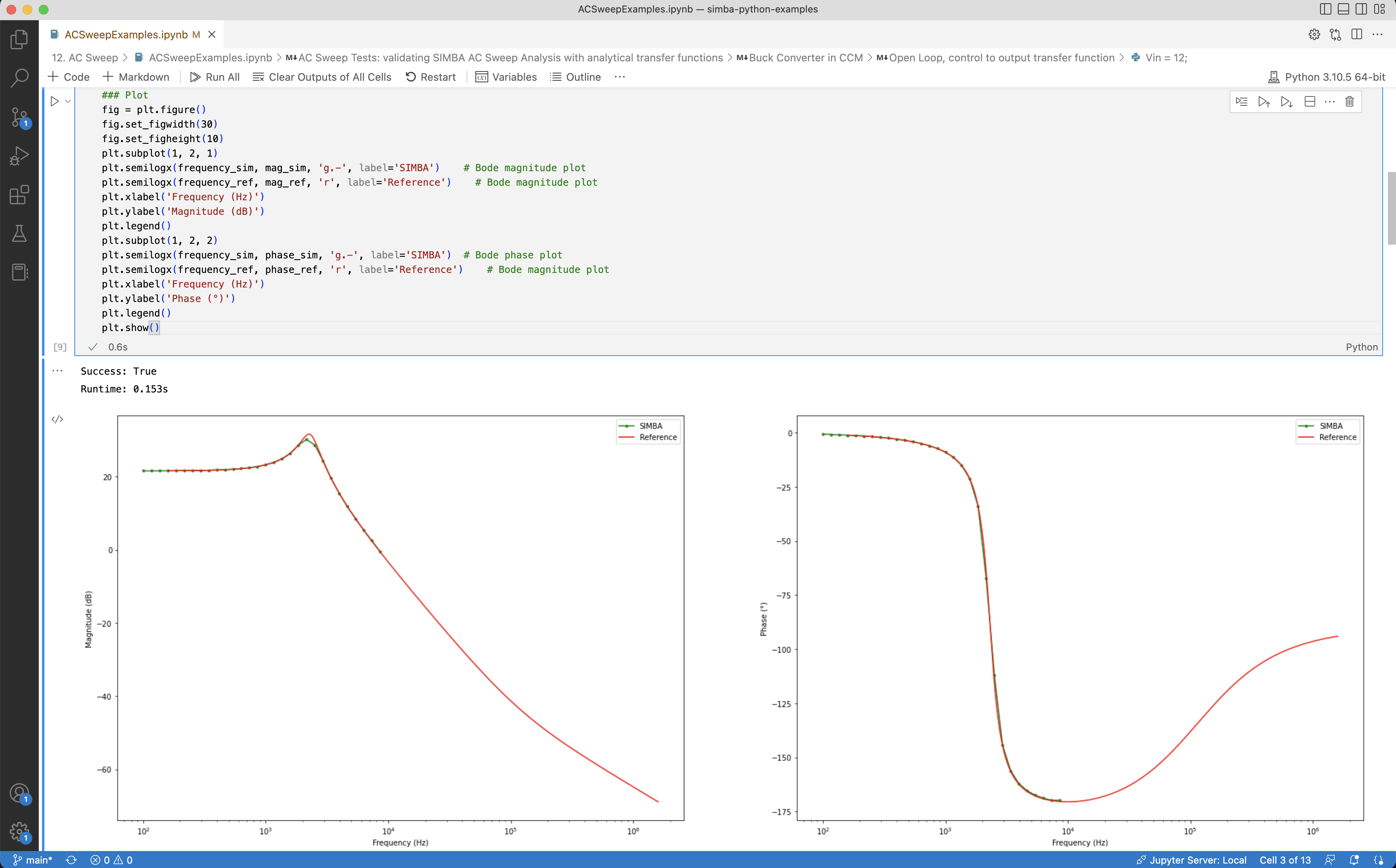# SIMBA Python Library

SIMBA python library is a Python package which allows the user to manage SIMBA (from circuit creation to simulation and post-processing) with Python scripts. As python syntax is very accessible, no python experience is required to use this library.

Thanks to the impressive number of modules for scientific computing, control and machine learning, python is a powerful tool.

With the SIMBA python library, it allows the user to integrate simba simulations and pre- and post-processing like never before: it can somehow replace the classic user files made with excel or Matlab® that come with the circuit simulation files!``````
from aesim.simba import Design
import matplotlib.pyplot as plt

# Create Design
design = Design()
design.Name = "DC/DC - Buck Converter"
design.TransientAnalysis.TimeStep = 1e-6
design.TransientAnalysis.EndTime = 10e-3
circuit = design.Circuit

V1 = circuit.AddDevice("DC Voltage Source", 2, 6)
V1.Voltage = 50
PWM = circuit.AddDevice("Square Wave", 2, 0)
PWM.Frequency = 5000
PWM.DutyCycle = 0.5
PWM.Amplitude = 1
D1.RotateLeft()
L1.Value = 1E-3
C1.RotateRight()
C1.Value = 100E-6
R1.RotateRight()
R1.Value = 5
R1.Name = "R1"
for scope in R1.Scopes:
scope.Enabled = True

# Make connections

#  Run Simulation
job = design.TransientAnalysis.NewJob()
status = job.Run()

# Get results
t = job.TimePoints
Vout = job.GetSignalByName('R1 - Instantaneous Voltage').DataPoints

# Plot Curve
fig, ax = plt.subplots()
ax.set_title(design.Name)
ax.set_ylabel('Vout (V)')
ax.set_xlabel('time (s)')
ax.plot(t,Vout)
``````

# Create and Modify Circuits

Circuits can be created from scratch and modified with a few command lines: adding, placing and connecting components are easily done.

# Transient, steady-state or AC analysis

Different circuit analysis can be performed to get transient or steady-state waveforms with respectively transient or steady-state analysis or to get transfer functions and impedances with the AC analysis.``````
import matplotlib.pyplot as plt
import numpy as np
from aesim.simba import DesignExamples

# Calculating Vout=f(dutycycle)
BuckBoostConverter = DesignExamples.BuckBoostConverter()
dutycycles = np.arange(0.00, 0.9, 0.9/100)
Vouts = []
for dutycycle in dutycycles:
# Set duty cycle value
PWM = BuckBoostConverter.Circuit.GetDeviceByName('C1')
PWM.DutyCycle=dutycycle

# Run calculation
job = BuckBoostConverter.TransientAnalysis.NewJob()
status = job.Run()

# Retrieve results
t = np.array(job.TimePoints)
Vout = np.array(job.GetSignalByName('R1 - Instantaneous Voltage').DataPoints)

# Average output voltage for t > 2ms
indices = np.where(t >= 0.002)
Vout = np.take(Vout, indices)
Vout = np.average(Vout)

# Save results
Vouts.append(Vout)

# Plot Curve
fig, ax = plt.subplots()
ax.set_title(BuckBoostConverter.Name)
ax.set_ylabel('Vout (V)')
ax.set_xlabel('Duty Cycle')
ax.plot(dutycycles,Vouts)
``````

# Parametric Analysis

Thanks to basic python functions such a 'FOR' loop, performing a parametric variation to get the influence of this parameters on the simulation is incredibly easy !